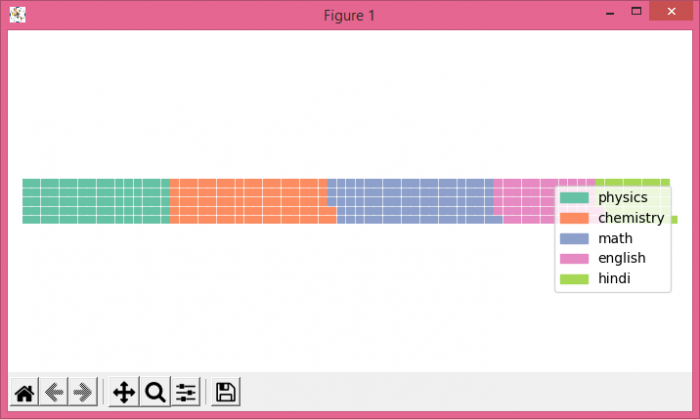# How to create waffle charts in Python Matplotlib?

A Waffle Chart is a gripping visualization technique that is normally created to display progress towards goals. While creating a new figure or activating an existing figure, we can use FigureClass=Waffle.

## Steps

• Create Panda's data frame using a dictionary.

• Create a new figure or activate an existing figure using FigureClass=Waffle, number of rows=5, values=df.price and labels=df.books.

• To display the figure, use show() method.

## Example

import pandas as pd
import matplotlib.pyplot as plt
from pywaffle import Waffle
plt.rcParams["figure.figsize"] = [7.00, 3.50]
plt.rcParams["figure.autolayout"] = True
data = {'books': ['physics', 'chemistry', 'math', 'english', 'hindi'],
'price': [80, 87, 89, 56, 39]
}
df = pd.DataFrame(data)
fig = plt.figure(
FigureClass=Waffle,
rows=5,
values=df.price,
labels=list(df.books)
)
plt.show()

## Output